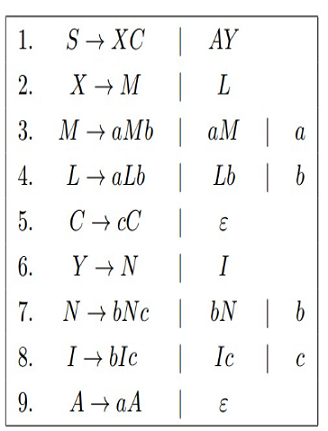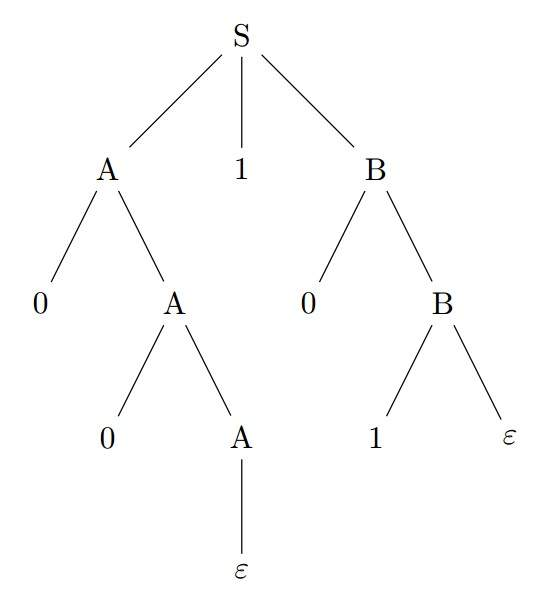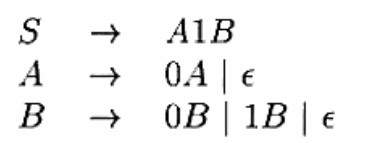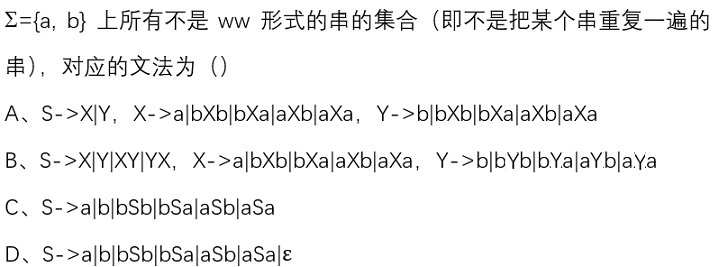# 第七章 上下文无关文法和推导--习题【含答案】 软件理论基础

（1）单选题

A  正确

B  错误

（2）单选题A  {a^i b^j c^k|i≠j or j≠k}

B  {a^i b^j c^k |i≠j or i≠k}

C  {a^i b^j c^k |i≠k or j≠k}

D  {a^i b^j c^k |i≠j and j≠k}

（3）单选题A  00101

B  0A101

C  A0B

D  A1B

（4）单选题A  S=>A1B=>1B=>10B=>100B=>1001B=>1001

B  S=>A1B=>A10B=>A100B=>A1001B=>A1001=>1001

C  S=>A1B=>1B=>10B=>1001B=>1001

D  S=>A1B=>A10B=>A100B=>A1001B=>1001

（5）单选题

A  5

B  6

C  4

D  7

（6）单选题A

B

C

D